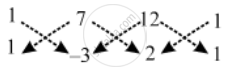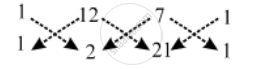Share

# Find the area of the quadrilateral ABCD whose vertices are respectively A(1, 1), B(7, –3), C(12, 2) and D(7, 21). - CBSE Class 10 - Mathematics

#### Question

Find the area of the quadrilateral ABCD whose vertices are respectively A(1, 1), B(7, –3), C(12, 2) and D(7, 21).

#### Solution

Area of quadrilateral ABCD = | Area of ∆ABC | + | Area of ∆ACD |

We have,∴ "Area of ∆ABC" = \frac { 1 }{ 2 } |(1× –3 + 7 × 2 + 12 × 1) – (7 × 1 + 12 × (–3) + 1× 2)|

"Area of ∆ABC" = \frac { 1 }{ 2 } |(–3 + 14 + 12) – (7 – 36 + 2)|

"Area of ∆ABC" = \frac { 1 }{ 2 } |23 + 27| = 25 sq. units

Also, we have"Area of ∆ACD" = \frac { 1 }{ 2 } |(1 ×2 + 12 × 21 + 7 × 1) – (12 × 1 + 7 × 2 + 1 × 21)|

"Area of ∆ACD" = \frac { 1 }{ 2 } |(2 + 252 + 7) – (12 + 14 + 21)|

"Area of ∆ACD" = \frac { 1 }{ 2 } |261 – 47| = 107 sq. units

Area of quadrilateral ABCD = 25 + 107 = 132 sq. units

Type III: On collinearity of three points

Three points A(x1 , y1 ), B(x2 , y2 ) and C(x3 , y3 ) are collinear iff

Area of ∆ABC = 0 i.e., x_1 (y_2 – y_3 ) + x_2 (y_3 – y_1 ) + x_3 (y_1 – y_2 ) = 0

Is there an error in this question or solution?

#### Video TutorialsVIEW ALL 

Solution Find the area of the quadrilateral ABCD whose vertices are respectively A(1, 1), B(7, –3), C(12, 2) and D(7, 21). Concept: Area of a Triangle.
S